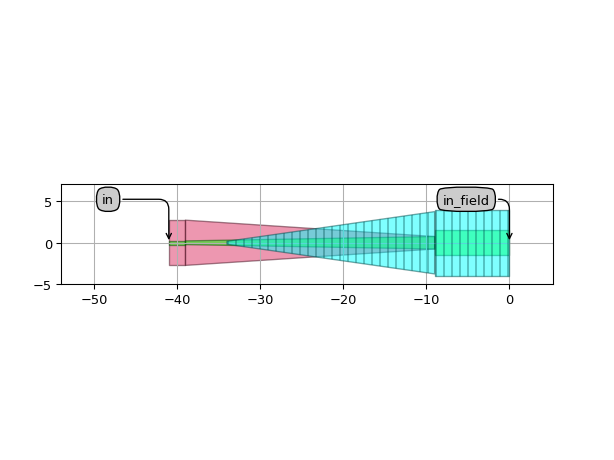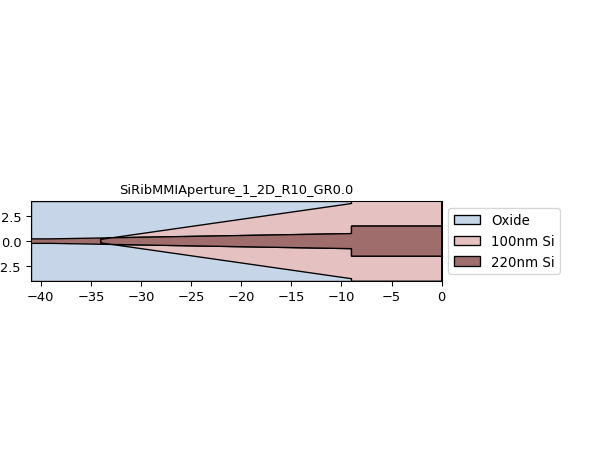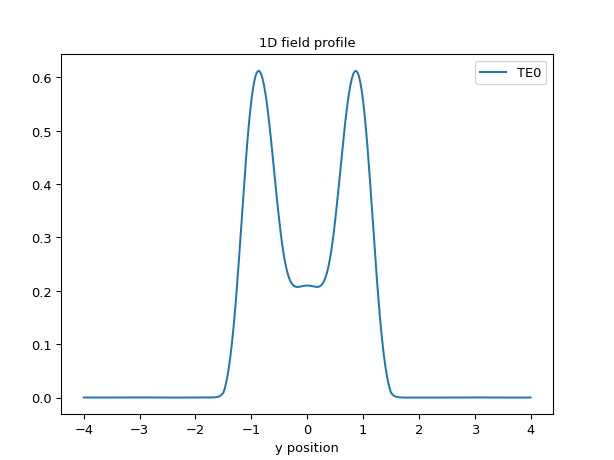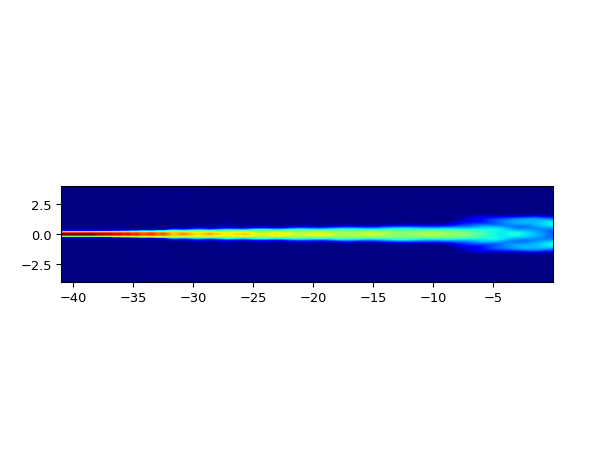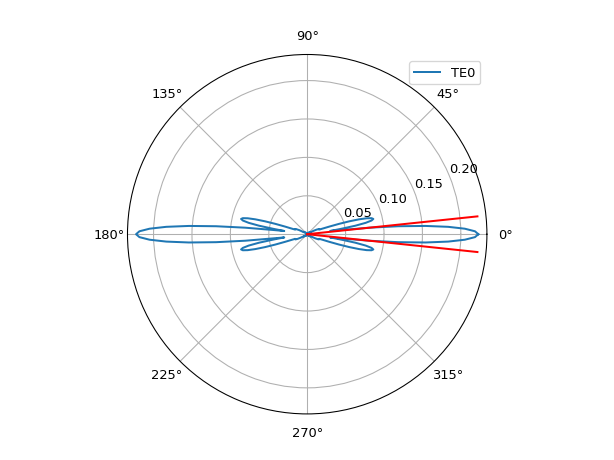# AWG components¶

The si_fab_awg module adds support for the IPKISS AWG Designer module. It defines technology-specific subcomponents and functions required for defining arrayed waveguide grating devices.

## SiSlabTemplate¶

A slab template defines the cross-section properties of the Free Propagation Region: the material stack and modes of the slab waveguide.

Reference

 si_fab_awg.all.SiSlabTemplate Silicon free propagation region slab waveguide template

Example

import si_fab.all as pdk
from si_fab_awg.all import SiSlabTemplate
import ipkiss3.all as i3
import numpy as np

wavelengths = np.linspace(1.5, 1.6, 101)

slab = SiSlabTemplate()

# visualize cross-section
slab_layout = slab.Layout()
slab_xs = slab_layout.cross_section()
slab_xs.visualize()

# visualize modes
slab_modes = slab.SlabModesFromCamfr()
slab_modes.visualize(wavelengths=wavelengths)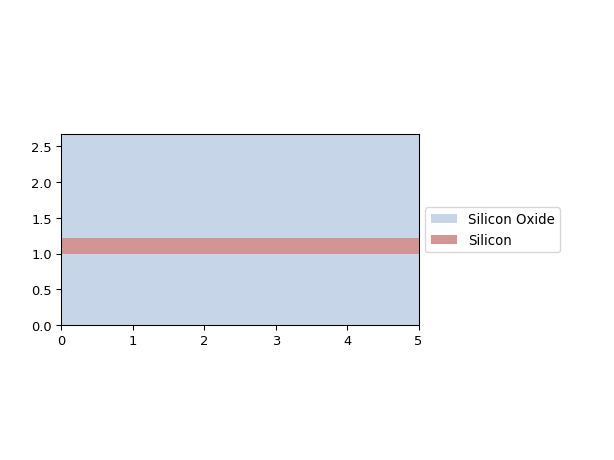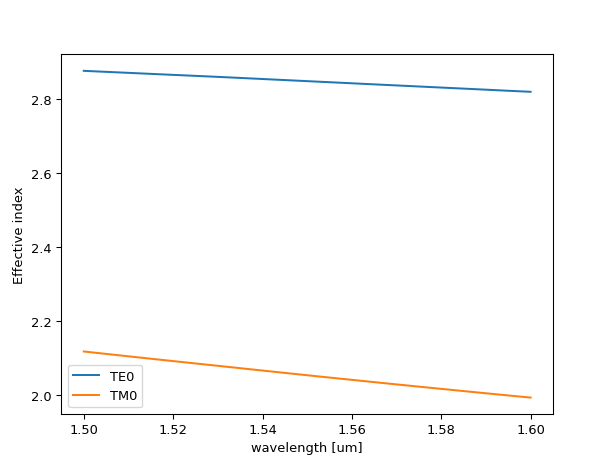## SiRibAperture¶

An aperture is a cell coupling light from an input waveguide into the Free Propagation Region. The field profiles at the aperture, that is at the point where the structure opens up into the Free Propagation Region, are used for simulating propagation through the star coupler.

Reference

 si_fab_awg.all.SiRibAperture Rib waveguide aperture into the silicon slab, with a strip waveguide start port.

Example

import si_fab.all as pdk
from si_fab_awg.all import SiSlabTemplate, SiRibAperture
import ipkiss3.all as i3
import numpy as np

wavelengths = np.linspace(1.5, 1.6, 100)
center_wavelength = 1.55

slab_tmpl = SiSlabTemplate()
slab_tmpl.SlabModesFromCamfr(wavelengths=wavelengths)

ap = SiRibAperture(slab_template=slab_tmpl)

ap_lay = ap.Layout()
ap_lay.visualize(annotate=True)
ap_lay.visualize_2d(process_flow=i3.TECH.VFABRICATION.PROCESS_FLOW_FEOL)

fieldmodel = ap.FieldModelFromCamfr()
env = i3.Environment(wavelength=center_wavelength)

# Field profile at the aperture
fields = fieldmodel.get_fields(mode=0, environment=env)
fields.visualize()

# Field propagated from input to the aperture
fields_2d = fieldmodel.get_aperture_fields2d(mode=0, environment=env)
fields_2d.visualize()

# Far field of the aperture
fields_ff = fieldmodel.get_far_field(mode=0, environment=env)
fields_ff.visualize()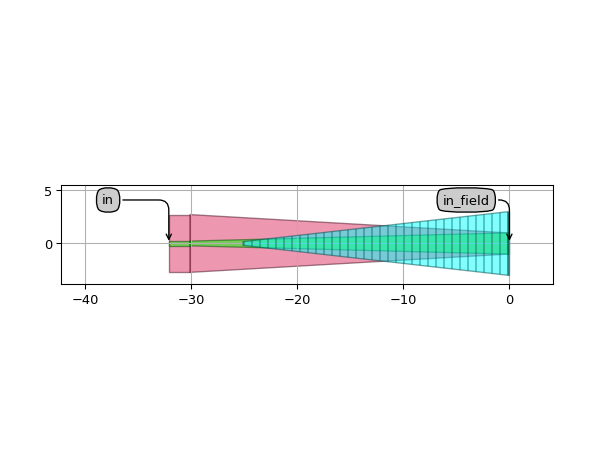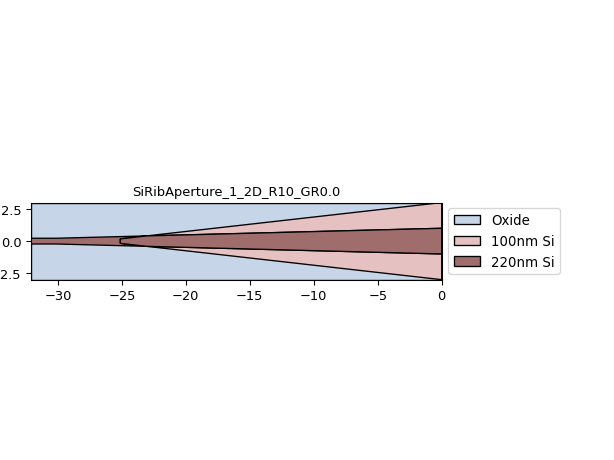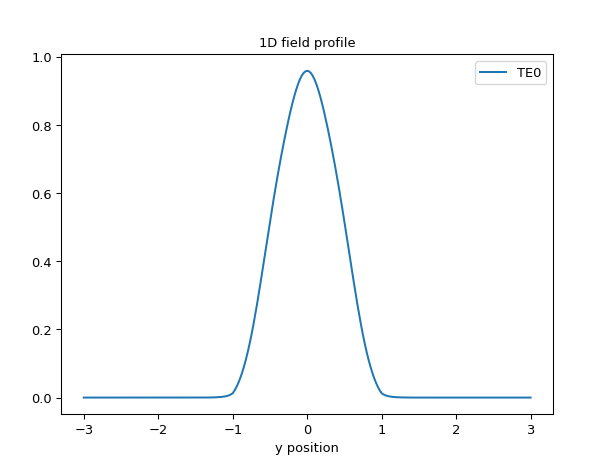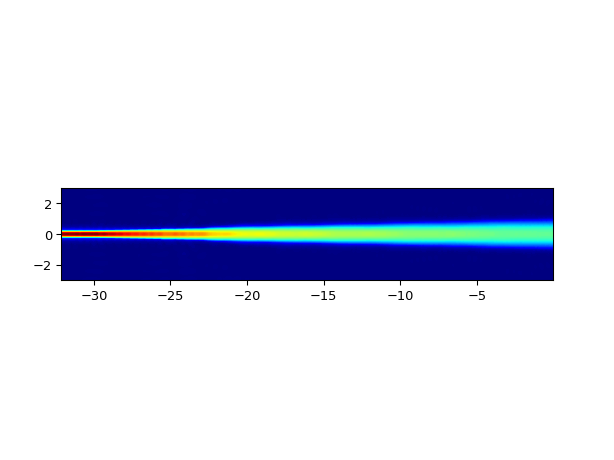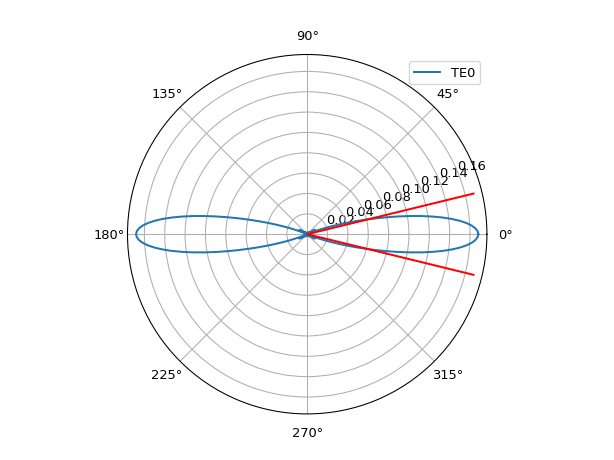## SiRibMMIAperture¶

The SiRibMMIAperture aperture couples light into the Free Propagation Region with a modified field profile consisting of 2 lobes.

Reference

 si_fab_awg.all.SiRibMMIAperture Multimode interferometric rib waveguide aperture into the silicon slab

Example

import si_fab.all as pdk
from si_fab_awg.all import SiSlabTemplate, SiRibMMIAperture
import ipkiss3.all as i3
import numpy as np

wavelengths = np.linspace(1.25, 1.35, 100)
center_wavelength = 1.3

slab_tmpl = SiSlabTemplate()
slab_tmpl.SlabModesFromCamfr(wavelengths=wavelengths)

ap = SiRibMMIAperture(slab_template=slab_tmpl,
mmi_core_width=3.0,
mmi_length=9.0,
taper_core_width=1.5,
taper_length=30.0)

# Layout
ap_lay = ap.Layout()
ap_lay.visualize(annotate=True)
ap_lay.visualize_2d(process_flow=i3.TECH.VFABRICATION.PROCESS_FLOW_FEOL)

# Field model
fieldmodel = ap.FieldModelFromCamfr()
env = i3.Environment(wavelength=center_wavelength)

# Field profile at the aperture
print("Simulating aperture field profile")
fields = fieldmodel.get_fields(mode=0, environment=env)
fields.visualize()

# Field propagated from input to the aperture
print("Simulating field through the full cell")
fields_2d = fieldmodel.get_aperture_fields2d(mode=0, environment=env)
fields_2d.visualize()

# Far field of the aperture
print("Calculating far field")
fields_ff = fieldmodel.get_far_field(mode=0, environment=env)
divergence_angle = fields_ff.divergence_angle(slab_mode='TE0', method='e_sq')
print(divergence_angle)
fields_ff.visualize()